# Unit 4 music theory intervals

Download 115.75 Kb.
 Page 1/2 Date conversion 30.04.2018 Size 115.75 Kb.
1   2

UNIT 4 MUSIC THEORY

INTERVALS

Music intervaldistance between two notes

Example: The distance between these notes is a 3rd.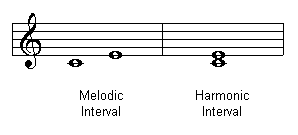How to count: Count the bottom note, and then all spaces and lines between and finish counting on the top note.

Two types of intervals:Melodic Interval: Two notes played separately at different times.

Harmonic Interval: Two notes played together at the same time.

Interval Quality

In a major scale, each note is an interval away from “Do”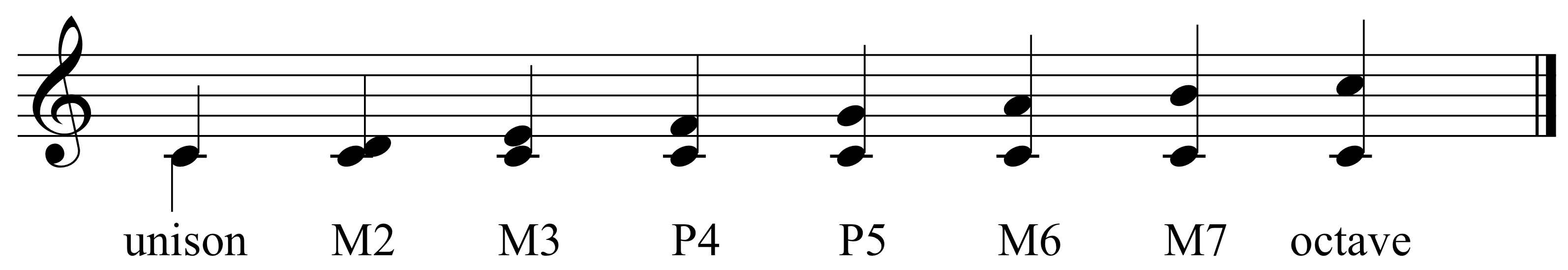Do Do –Re Do-Mi Do-Fa Do – Sol Sol – La Do- Ti Do – Do

Do to (same note) Do is a “Perfect Unison” or “P1”

Do to High Doe is a “Perfect Octave” or “P8”

You need to memorize the pattern above

It doesn’t matter if Do starts at on “C” or “D” or “F” or any letter, the pattern is the same.

FIGURING OUT INTERVAL QUALITY

EXAMPLE 1: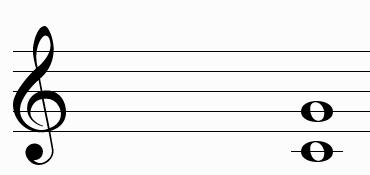Step 1 – label the bottom note as “do”

Example: Do = C

Step 2 – Determine what “do’s” key signature looks like

Example: C has no sharps and no flats

Step 3 – Write out the scale using the key signature

Example: C major Scale: C, D, E, F, G, A, B, C

Step 4 – Determine the top note letter name

Example: G

Step 5 – Determine if the top note is in the scale

Example: G is in the scale

Step 6 – If it is in the scale then refer to your pattern: P1, M2, M3, P4, P5, M6, M7, P8 and label the quality

Example: P5 is the answer

EXAMPLE 2: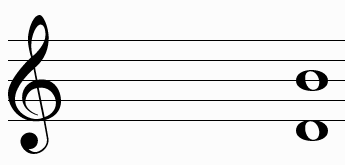Step 1 – label the bottom note as “do”

Example: Do = D

Step 2 – Determine what “do’s” key signature looks like

Example: D has 2 sharps, F# & C#

Step 3 – Write out the scale using the key signature

Example: D major Scale: D, E, F#, G, A, B, C#, D

Step 4 – Determine the top note letter name

Example: B

Step 5 – Determine if the top note is in the scale

Example: B is in the scale

Step 6 – If it is in the scale then refer to your pattern: P1, M2, M3, P4, P5, M6, M7, P8 and label the quality

Example: M6 is the answer

EXAMPLE 3: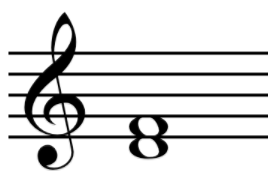Step 1 – label the bottom note as “do”

Example: Do = D

Step 2 – Determine what “do’s” key signature looks like

Example: D has 2 sharps, F# & C#

Step 3 – Write out the scale using the key signature

Example: D major Scale: D, E, F#, G, A, B, C#, D

Step 4 – Determine the top note letter name

Example: F

Step 5 – Determine if the top note is in the scale

Example: F is in the scale – it is F# in the scale

Step 6 – If it is NOT in the scale then refer to your pattern: P1, M2, M3, P4, P5, M6, M7, P8 and refer to your chart here:

 Major Intervals Perfect Intervals Augmented Augmented Major Perfect minor diminished Diminished
1   2

The database is protected by copyright ©ininet.org 2016
send message